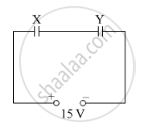# Calculate Capacitance of Each Capacitor If the Equivalent Capacitance of the Combination is 4 μF. - Physics

Two parallel-plate capacitors X and Y have the same area of plates and same separation between them. X has air between the plates, while Y contains a dielectric medium of εr = 4.(i) Calculate capacitance of each capacitor if the equivalent capacitance of the combination is 4 μF.

(ii) Calculate the potential difference between the plates X and Y.

(iii) Estimate the ratio of electrostatic energies stored in X and Y.

#### Solution

(i) We know that the capacitance of a parallel-plate capacitor is given by

C=(varepsilon_0varepsilon_rA)/d

C_Y=(varepsilon_0 4A)/d

C_X=(varepsilon_0 A)/d

Thus,

=>C_Y=4C_X

=>C_(eq)=(C_xC_Y)/(C_x+C_Y) = 4muF

=>(C_X(4C_X))/(5C_X)=4muF

=>C_X=5muF

=>C_Y=20muF

(ii) The potential difference between plates X and Y can be calculated as follows:

Q=CV

=>C_XV_X=C_YV_Y

=>V_X/V_Y=C_X/C_Y=4

=>V_X=4V_Y

Also, VY+VX=15

VY=3 V

VX=12 V

(iii) The ratio of electrostatic energies can be calculated as follows:

E=(Q^2)/(2C)

=>E_X/E_Y=C_Y/C_X=4

=>E_X/E_Y=4/1

Concept: Capacitance of a Parallel Plate Capacitor with and Without Dielectric Medium Between the Plates
Is there an error in this question or solution?